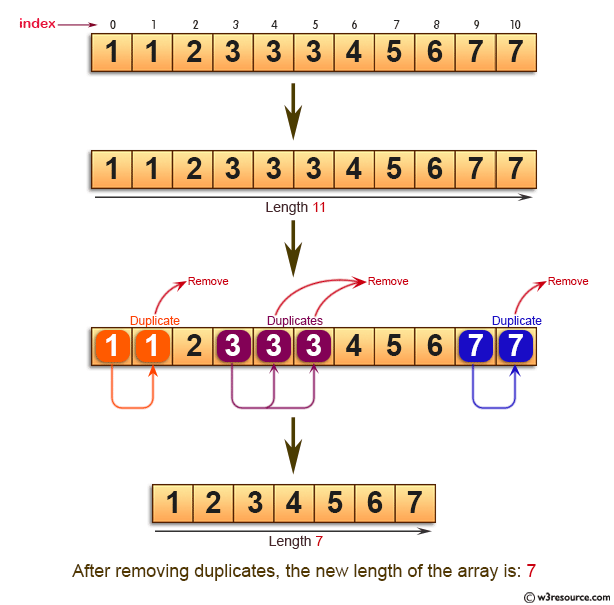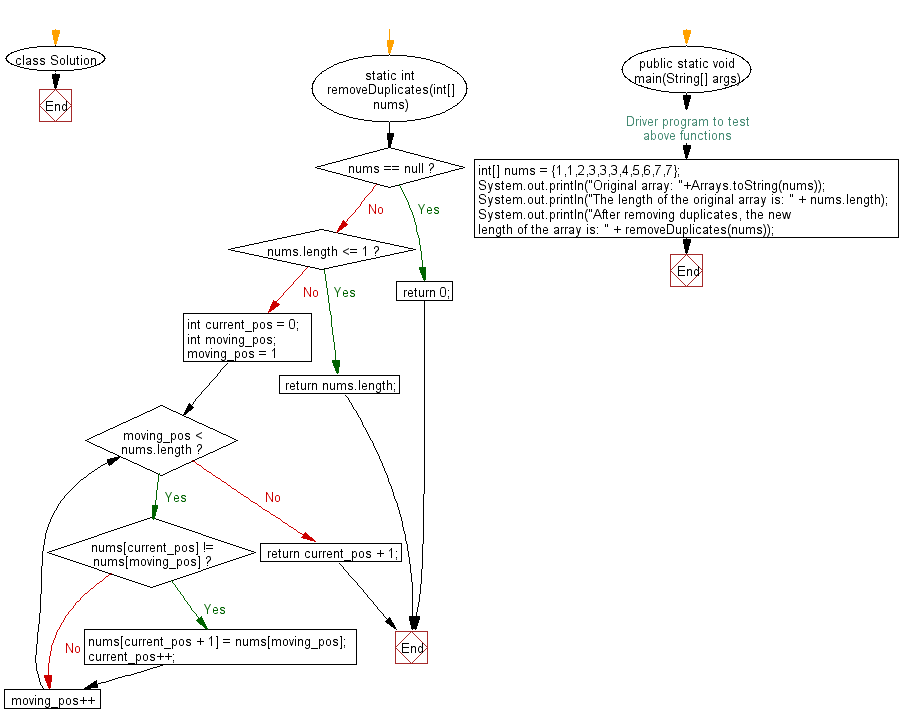﻿ Java: Length of a array where each value appear only once# Java Exercises: Find the new length of a given sorted array where each element appear only once

## Java Basic: Exercise-131 with Solution

Write a Java program to find the new length of a given sorted array where each element appear only once (remove the duplicates ).

Pictorial Presentation:Sample Solution:

Java Code:

``````import java.util.Arrays;
class Solution {
static  int removeDuplicates(int[] nums) {
if (nums == null) {
return 0;
}
if (nums.length <= 1) {
return nums.length;
}
int current_pos = 0;
int moving_pos;
for (moving_pos = 1; moving_pos < nums.length; moving_pos++) {
if (nums[current_pos] != nums[moving_pos]) {
nums[current_pos + 1] = nums[moving_pos];
current_pos++;
}
}
return current_pos + 1;
}

/* Driver program to test above functions */
public static void main(String[] args)
{
int[] nums = {1,1,2,3,3,3,4,5,6,7,7};
System.out.println("Original array: "+Arrays.toString(nums));
System.out.println("The length of the original array is: " + nums.length);
System.out.println("After removing duplicates, the new length of the array is: " + removeDuplicates(nums));
}
}
```
```

Sample Output:

```Original array: [1, 1, 2, 3, 3, 3, 4, 5, 6, 7, 7]
The length of the original array is: 11
After removing duplicates, the new length of the array is: 7
```

Flowchart:Java Code Editor:

What is the difficulty level of this exercise?

Test your Programming skills with w3resource's quiz.

﻿

## Java: Tips of the Day

getEnumMap

Converts to enum to Map where key is the name and value is Enum itself.

```public static <E extends Enum<E>> Map<String, E> getEnumMap(final Class<E> enumClass) {
return Arrays.stream(enumClass.getEnumConstants())
.collect(Collectors.toMap(Enum::name, Function.identity()));
}
```

Ref: https://bit.ly/3xXcFZt